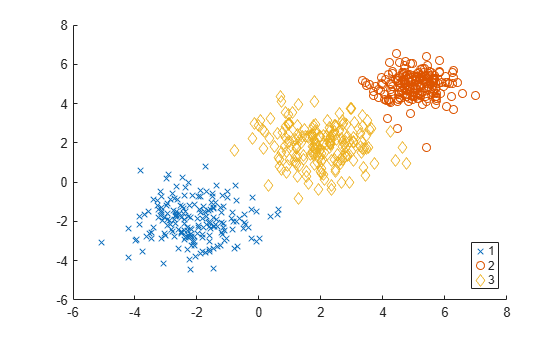# SilhouetteEvaluation

Silhouette criterion clustering evaluation object

## Description

`SilhouetteEvaluation` is an object consisting of sample data (`X`), clustering data (`OptimalY`), and silhouette criterion values (`CriterionValues`) used to evaluate the optimal number of data clusters (`OptimalK`). The silhouette value for each point (observation in `X`) is a measure of how similar that point is to other points in the same cluster, compared to points in other clusters. If most points have a high silhouette value, then the clustering solution is appropriate. If many points have a low or negative silhouette value, then the clustering solution might have too many or too few clusters. For more information, see Silhouette Value and Criterion.

## Creation

Create a silhouette criterion clustering evaluation object by using the `evalclusters` function and specifying the criterion as `"silhouette"`.

You can then use `compact` to create a compact version of the silhouette criterion clustering evaluation object. The function removes the contents of the properties `X`, `OptimalY`, and `Missing`.

## Properties

expand all

### Clustering Evaluation Properties

Clustering algorithm used to cluster the sample data, returned as `'kmeans'`, `'linkage'`, `'gmdistribution'`, or a function handle. If you specify the clustering solutions as an input argument to `evalclusters` when you create the clustering evaluation object, then `ClusteringFunction` is empty.

ValueDescription
`'kmeans'`Cluster the data in `X` using the `kmeans` clustering algorithm, with `EmptyAction` set to `"singleton"` and `Replicates` set to `5`.
`'linkage'`Cluster the data in `X` using the `clusterdata` agglomerative clustering algorithm, with `Linkage` set to `"ward"`.
`'gmdistribution'`Cluster the data in `X` using the `gmdistribution` Gaussian mixture distribution algorithm, with `SharedCov` set to `true` and `Replicates` set to `5`.

Data Types: `double` | `char` | `function_handle`

Prior probabilities for each cluster, returned as `'empirical'` or `'equal'`.

ValueDescription
`'empirical'`Compute the silhouette criterion value for the clustering solution by averaging the silhouette values for all points. Each cluster contributes to the criterion value proportionally based on its size.
`'equal'`Compute the silhouette criterion value for the clustering solution by averaging the silhouette values for all points within each cluster, and then averaging those values across all clusters. Regardless of its size, each cluster contributes equally to the criterion value.

Average silhouette values corresponding to each proposed number of clusters in `InspectedK`, returned as a cell array of numeric vectors. For each proposed number of clusters `k`, the vector `ClusterSilhouettes{k}` contains the average silhouette value for each cluster.

For example, suppose `evaluation` is a silhouette criterion clustering evaluation object and `evaluation.InspectedK` is `1:5`. Then, `evaluation.ClusterSilhouettes{4}(3)` is the average silhouette value for the points in the third cluster of the clustering solution with four total clusters.

Data Types: `cell`

Name of the criterion used for clustering evaluation, returned as `'Silhouette'`.

Criterion values, returned as a numeric vector. Each value corresponds to a proposed number of clusters in `InspectedK`.

Data Types: `double`

Distance metric used for clustering data and computing the criterion values, returned as one of the values in this table, a function handle, or a numeric vector returned by the function `pdist`.

ValueDescription
`'sqEuclidean'`Squared Euclidean distance
`'Euclidean'`Euclidean distance
`'cityblock'`Sum of absolute differences
`'cosine'`One minus the cosine of the included angle between points (treated as vectors)
`'correlation'`One minus the sample correlation between points (treated as sequences of values)
`'Hamming'`Percentage of coordinates that differ
`'Jaccard'`Percentage of nonzero coordinates that differ

Data Types: `single` | `double` | `char` | `function_handle`

List of the number of proposed clusters for which to compute criterion values, returned as a positive integer vector.

Data Types: `double`

Optimal number of clusters, returned as a positive integer scalar.

Data Types: `double`

Optimal clustering solution corresponding to `OptimalK`, returned as a positive integer column vector. Each row of `OptimalY` represents the cluster index of the corresponding observation (or row) in `X`. If you specify the clustering solutions as an input argument to `evalclusters` when you create the clustering evaluation object, or if the clustering evaluation object is compact (see `compact`), then `OptimalY` is empty.

Data Types: `double`

### Sample Data Properties

Excluded data, returned as a logical column vector. If an element of `Missing` is `true`, then the corresponding observation (or row) in the data matrix `X` is not used in the clustering solutions. If the clustering evaluation object is compact (see `compact`), then `Missing` is empty.

Data Types: `double` | `logical`

Number of observations in the data matrix `X`, ignoring observations with missing (`NaN`) values, returned as a positive integer scalar.

Data Types: `double`

Data used for clustering, returned as a numeric matrix. Rows correspond to observations, and columns correspond to variables. If the clustering evaluation object is compact (see `compact`), then `X` is empty.

Data Types: `single` | `double`

## Object Functions

 `addK` Evaluate additional numbers of clusters `compact` Compact clustering evaluation object `plot` Plot clustering evaluation object criterion values

## Examples

collapse all

Evaluate the optimal number of clusters using the silhouette clustering evaluation criterion.

Generate sample data containing random numbers from three multivariate distributions with different parameter values.

```rng("default") % For reproducibility n = 200; mu1 = [2 2]; sigma1 = [0.9 -0.0255; -0.0255 0.9]; mu2 = [5 5]; sigma2 = [0.5 0; 0 0.3]; mu3 = [-2 -2]; sigma3 = [1 0; 0 0.9]; X = [mvnrnd(mu1,sigma1,n); ... mvnrnd(mu2,sigma2,n); ... mvnrnd(mu3,sigma3,n)];```

Evaluate the optimal number of clusters using the silhouette criterion. Cluster the data using `kmeans`.

`evaluation = evalclusters(X,"kmeans","silhouette","KList",1:6)`
```evaluation = SilhouetteEvaluation with properties: NumObservations: 600 InspectedK: [1 2 3 4 5 6] CriterionValues: [NaN 0.8055 0.8551 0.7155 0.6071 0.6232] OptimalK: 3 ```

The `OptimalK` value indicates that, based on the silhouette criterion, the optimal number of clusters is three.

Plot the silhouette criterion values for each number of clusters tested.

`plot(evaluation)`The plot shows that the highest silhouette value occurs at three clusters, suggesting that the optimal number of clusters is three.

Create a grouped scatter plot to visually examine the suggested clusters.

```clusters = evaluation.OptimalY; gscatter(X(:,1),X(:,2),clusters,[],"xod")```The plot shows three distinct clusters within the data: cluster 1 in the lower-left corner, cluster 2 in the upper-right corner, and cluster 3 near the center of the plot.